Latest Banking jobs   »   Reasoning Ability Quiz For SBI PO/Clerk...

# Reasoning Ability Quiz For SBI PO/Clerk Mains 2023- 8th January

Directions (1-3): Each of the questions below consists of a question and two statements numbered I and II given below it. You have to decide whether the data provided in the statements are sufficient to answer the question. Read both the statements and give answer-

Q1. Five friends Q, R, S, T, V are born on different dates 10, 18, 21, 26, 29 (but not necessarily in the same order) of the month of January and September on the same year. V is born on which of the following date of which month?
(I) Only two persons born between Q and V. Both Q and V born on the even date. R born after S. Not more than three persons born in the same month. R born on the odd prime number date.
(II) No one born after T. R born on the odd date of the month having 31 days. R born before V.
(a) if the data in statement I alone are sufficient to answer the question, while the data in statement II alone are not sufficient to answer the question.
(b) if the data in statement II alone are sufficient to answer the question, while the data in statement I alone are not sufficient to answer the question.
(c) if the data either in statement I alone or in statement II alone are sufficient to answer the question.
(d) if the data even in both statements I and II together are not sufficient to answer the question.
(e) if the data in both statements I and II together are necessary to answer the question.

Q2. Six boxes M, N, O, P, Q, R of different colours is placed one above another. Also, each box has different number of toffees. No two boxes have same number of toffees. Only two boxes are kept between Box O and Blue colour box. No box is kept below Blue colour box. Only one box is kept between Box R and Green colour box. Box R is kept either immediately below or immediately above box O. Box R is kept below Green colour box. Box M is of Yellow colour. Box M is kept immediately above box Q, which is kept above box N. Only one box is kept between Box N and Red colour. Box P is not of White colour. Only one box kept between Black colour box and box P. Box R is not of Red colour. How many numbers of toffees does red box have?
(I) Box M has 8 less Toffees than Blue colour box. The box having lowest and second lowest number of toffees box have 10 and 11 toffees respectively. Black box has 4 less number of toffees than box P and 3 more number of toffees than Box N.
(II) Q and M are the boxes having lowest and 2nd lowest number of toffees in them. Box O has 5 more number of toffees than white colour box.

(a) if the data in statement I alone are sufficient to answer the question, while the data in statement II alone are not sufficient to answer the question.
(b) if the data in statement II alone are sufficient to answer the question, while the data in statement I alone are not sufficient to answer the question.
(c) if the data either in statement I alone or in statement II alone are sufficient to answer the question.
(d) if the data even in both statements I and II together are not sufficient to answer the question.
(e) if the data in both statements I and II together are necessary to answer the question.

Q3. On which of the following day of the week (starting from Monday and ends on Sunday) E was born?
I. Only three persons born between C and D. C born before D. F is born either immediately before or immediately after C.
II. Only two persons born between F and A. E born before G. B born before A but not immediately before.
(a) if the data in statement I alone are sufficient to answer the question, while the data in statement II alone are not sufficient to answer the question.
(b) if the data in statement II alone are sufficient to answer the question, while the data in statement I alone are not sufficient to answer the question.
(c) if the data either in statement I alone or in statement II alone are sufficient to answer the question.
(d) if the data even in both statements I and II together are not sufficient to answer the question.
(e) if the data in both statements I and II together are necessary to answer the question.

Directions (4–5): In each question below is given a statement followed by three courses of action numbered I, II and III. A course of action is a step or administrative decision to be taken for improvement, follow-up or further action in regard to the problems, policy etc. on the basis of the information given in the statement, you have to assume everything in the statement to be true, then decide of the suggested courses of action logically follows for pursuing.

Q4. The major road connecting the two main parts of the city is inundated due to heavy rains during past two days.
Courses of Action:
(I)The govt. should immediately send a relief team to the affected area.
(II)The municipal authority should make immediate effort to pump out water from the road.
(III)The municipal authority should advise the general public to stay indoors till the water is cleared.
(a) Only I follows
(b) Only II follow.
(c) Only I and III follow.
(d) Only II and III follow.
(e) None of these

Q5. The prices of essential commodities have gone up substantially during the past few weeks.
Courses of Action:
(I)The government should set up an expert committee to study the trend of prices.
(II)The govt. should immediately abolish taxes on essential commodities.
(III)The govt. should advise the general public to refrain from purchasing essential commodities for few days.
(a) None of these
(b) Only I follows.
(c) Only II follows.
(d) Only III follows.
(e) Only I and II follow.

Directions (6-8): Study the following information carefully to answer the given questions:
There are six employees A, B, C, D, E, F of a company and all of them are working on six different designations of a company viz. CMD, MD, CEO, COO, SE, JE. All the designations given are to be considered in a given order (as CMD is considered as Senior-most and JE is considered as the Junior-most).
The one who likes Orchid is junior than B. D does not like Orchid and Lily. One of the persons likes Lotus. E is just junior than the one who likes Lily. Only two persons are junior than D. The one who likes Rose is junior to only one person. A likes Tulip and is Senior than B. E is senior than F. B does not like Sunflower. A is not SE. B does not like Rose.

Q6. Who among the following is SE of the company?
(a) C
(b) A
(c) B
(d) D
(e) F

Q7. Who among the following likes Rose?
(a) E
(b) C
(c) A
(d) B
(e) D

Q8. How many persons are junior than the one who likes Tulip?
(a) One
(b) Two
(c) Four
(d) Three
(e) None of these

Directions (9-10): Study the following information carefully and answer the questions given below:
Seven friends J, K, L, M, N, O and P are sitting in a garden at different positions. J is 13 meters North of N, who is 25 meters east of K. M is 5 meters Southeast of L. P is East of L. The distance between P and L is same as distance between J and N. O is 7 meters North of K. M is west of J. P is 3 meters North of J.

Q9. What is the shortest distance between L and O?
(a) 12 meters
(b) 10 meters
(c) 15 meters
(d) 17 meters
(e) 13 meters

Q10. If M moves 13meters South then what is the distance between K and M?
(a) 12 meters
(b) 14 meters
(c) 15 meters
(d) 16 meters
(e) 9 meters

Directions (11-13): These questions are based on the following information.
‘P@Q’ means ‘P is the mother of Q’
‘P\$Q’ means ‘P is the husband of Q’
‘P#Q’ means ‘P is the sister of Q’
‘P*Q’ means ‘P is the son of Q’.

Q11. Which of the following indicates the relationship ‘F is the son of A?
(a) A#R#F*B@T
(b) R#A\$F@B\$T
(c) T#B#R*F\$A
(d) T@B#R*F*A
(e) None of these

Q12. If the expressions J#P\$Z and P*B\$K, are true, then how is Z related to K?
(a) Mother
(b) Father
(c) Daughter-in-law
(d) Sister
(e) None of these

Q13. If K#O*Q\$M@N, then which of the following is definitely true?
(a) M is the sister of K
(b) K is the father of N
(c) M is the father of K
(d) O is the brother of K
(e) None of these

Directions (14-15): In the following questions, the symbols #, &, @ and \$ are used with the following meanings as illustrated below. Study the following information and answer the given questions:
Note: The directions which are given indicates exact directions.
P#Q – Q is in the south direction of P.
P@Q – Q is in the north direction of P
P&Q – Q is in the east direction of P.
P\$Q – Q is in the west direction of P.
P#&Q – Q or P is in the southeast direction of P or Q.
P@&Q – P or Q is in the northeast direction of Q or P.

Q14. If A#B\$C@&D@E are related to each other such that F is in west of E and also the midpoint of A and B. What is the shortest distance between C and E?
Note: BC=EF=4m, 4DE=AB=12m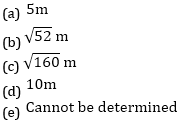Q15. If A#B\$C@&D@E\$F@&G are related to each other such that F and G are the midpoint of line AB and BC respectively. Then what is the relation between G and D?
Note: 4DE=AB=12m
(a) D#G
(b) D#&G
(c) G@&D
(d) G&D
(e) Cannot be determined

Solutions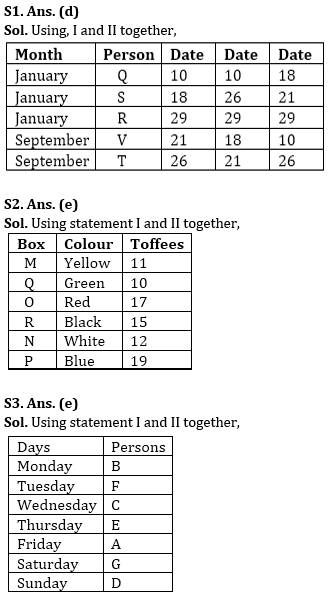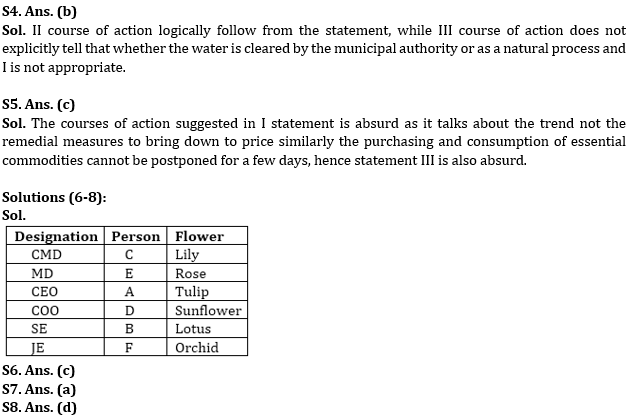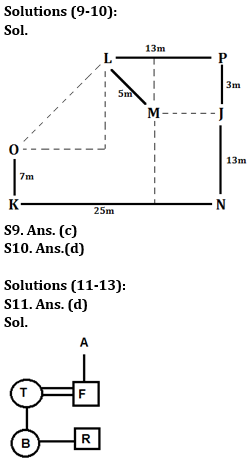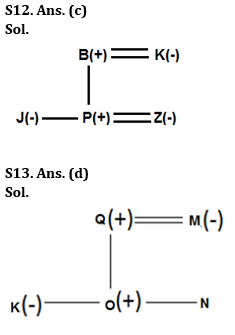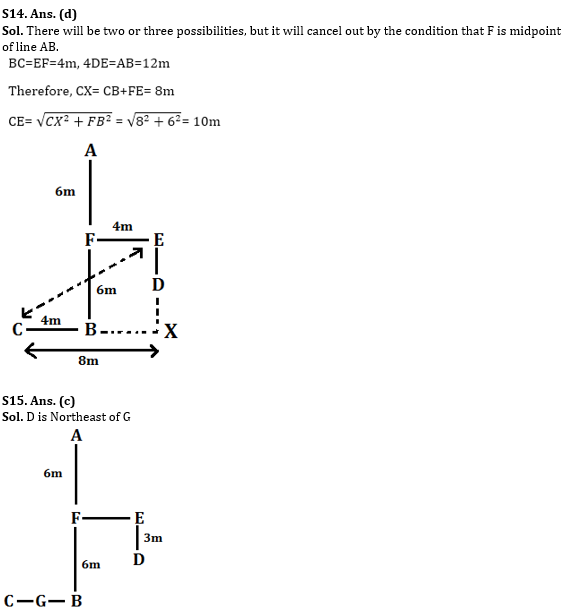## FAQs

### Q1. Five friends Q, R, S, T, V are born on different dates 10, 18, 21, 26, 29 (but not necessarily in the same order) of the month of January and September on the same year. V is born on which of the following date of which month?

S1. Ans. (d)

#### Congratulations!